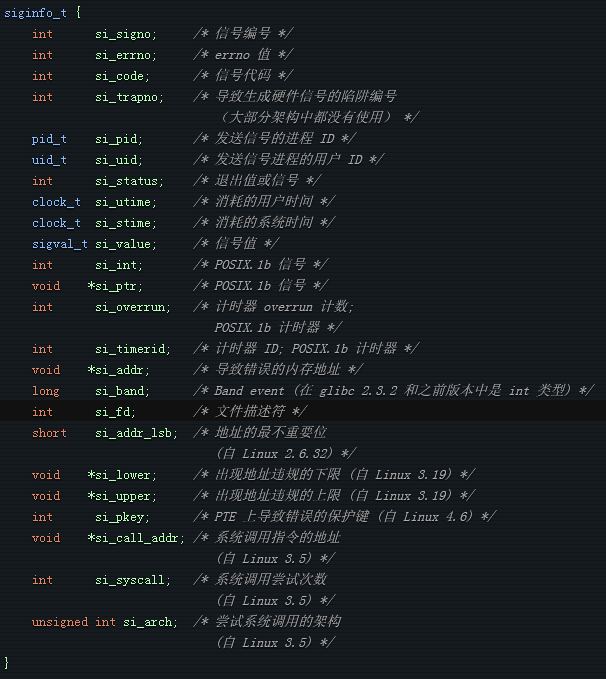# 开发一个Linux调试器（五）：源码和信号

class debugger {
public:
debugger (std::string prog_name, pid_t pid)
: m_prog_name{std::move(prog_name)}, m_pid{pid} {
auto fd = open(m_prog_name.c_str(), O_RDONLY);
m_elf = elf::elf{elf::create_mmap_loader(fd)};
m_dwarf = dwarf::dwarf{dwarf::elf::create_loader(m_elf)};
}
//...
private:
//...
dwarf::dwarf m_dwarf;
elf::elf m_elf;
};

dwarf::die debugger::get_function_from_pc(uint64_t pc) {
for (auto &cu : m_dwarf.compilation_units()) {
if (die_pc_range(cu.root()).contains(pc)) {
for (const auto& die : cu.root()) {
if (die.tag == dwarf::DW_TAG::subprogram) {
if (die_pc_range(die).contains(pc)) {
return die;
}
}
}
}
}
throw std::out_of_range{"Cannot find function"};
}

dwarf::line_table::iterator debugger::get_line_entry_from_pc(uint64_t pc) {
for (auto &cu : m_dwarf.compilation_units()) {
if (die_pc_range(cu.root()).contains(pc)) {
auto &lt = cu.get_line_table();
auto it = lt.find_address(pc);
if (it == lt.end()) {
throw std::out_of_range{"Cannot find line entry"};
}
else {
return it;
}
}
}
throw std::out_of_range{"Cannot find line entry"};
}

void debugger::print_source(const std::string& file_name, unsigned line, unsigned n_lines_context) {
std::ifstream file {file_name};
//获得一个所需行附近的窗口
auto start_line = line <= n_lines_context ? 1 : line - n_lines_context;
auto end_line = line + n_lines_context + (line < n_lines_context ? n_lines_context - line : 0) + 1;
char c{};
auto current_line = 1u;
//跳过 start_line 之前的行
while (current_line != start_line && file.get(c)) {
if (c == '\n') {
++current_line;
}
}
//如果我们在当前行则输出光标
std::cout << (current_line==line ? "> " : "  ");
//输出行直到 end_line
while (current_line <= end_line && file.get(c)) {
std::cout << c;
if (c == '\n') {
++current_line;
//如果我们在当前行则输出光标
std::cout << (current_line==line ? "> " : "  ");
}
}
//输出换行确保恰当地清空了流
std::cout << std::endl;
}

siginfo_t debugger::get_signal_info() {
siginfo_t info;
ptrace(PTRACE_GETSIGINFO, m_pid, nullptr, &info);
return info;
}void debugger::wait_for_signal() {
int wait_status;
auto options = 0;
waitpid(m_pid, &wait_status, options);
auto siginfo = get_signal_info();
switch (siginfo.si_signo) {
case SIGTRAP:
handle_sigtrap(siginfo);
break;
case SIGSEGV:
std::cout << "Yay, segfault. Reason: " << siginfo.si_code << std::endl;
break;
default:
std::cout << "Got signal " << strsignal(siginfo.si_signo) << std::endl;
}
}

void debugger::handle_sigtrap(siginfo_t info) {
switch (info.si_code) {
//如果命中了一个断点其中的一个会被设置
case SI_KERNEL:
case TRAP_BRKPT:
{
set_pc(get_pc()-1); //将程序计数器的值设置为它应该指向的地方
std::cout << "Hit breakpoint at address 0x" << std::hex << get_pc() << std::endl;
auto line_entry = get_line_entry_from_pc(get_pc());
print_source(line_entry->file->path, line_entry->line);
return;
}
//如果信号是由逐步执行发送的，这会被设置
case TRAP_TRACE:
return;
default:
std::cout << "Unknown SIGTRAP code " << info.si_code << std::endl;
return;
}
}

void debugger::step_over_breakpoint() {
if (m_breakpoints.count(get_pc())) {
auto& bp = m_breakpoints[get_pc()];
if (bp.is_enabled()) {
bp.disable();
ptrace(PTRACE_SINGLESTEP, m_pid, nullptr, nullptr);
wait_for_signal();
bp.enable();
}
}
}